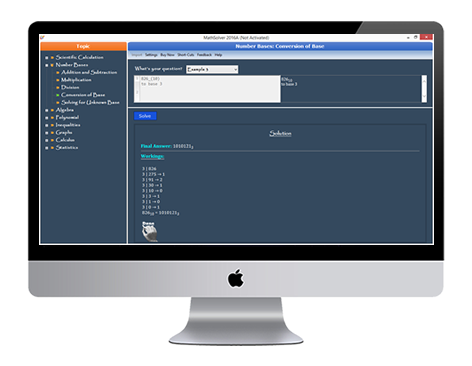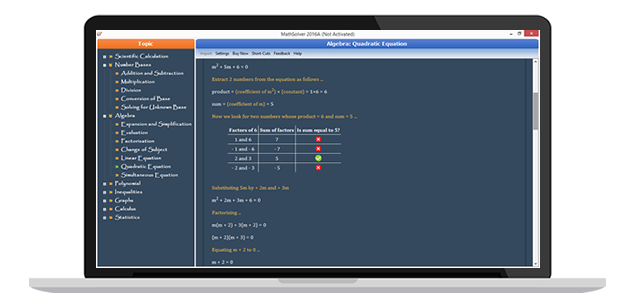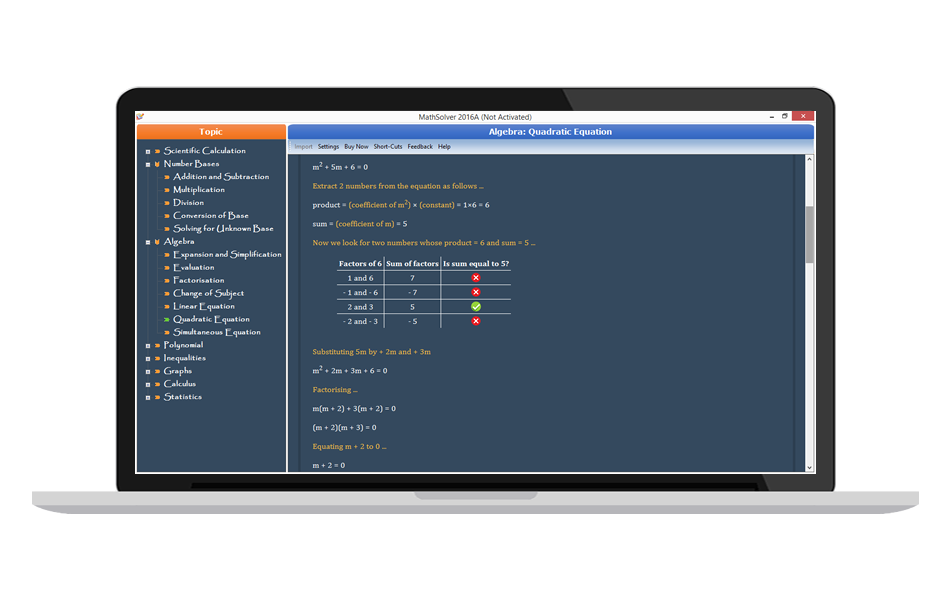## MathSolver

MathSolver helps to solve mathematical problems provided by the user. It provides adequate workings to support answers; and this happens on-the-fly

MathSolver helps to solve mathematical problems within a set of topics provided by the user. It provides adequate workings to support answers; and this happen on-the-fly. It has 8 topics, 34 sub-topics and 234 illustrative examples.• As a scientific calculator
• To learn a topic in Math from the following topics , Number Bases, Algebra, Polynomials, Inequalities, Graphs, Calculus, Statistics.
• To get detailed workings to exercises or assignment
• To verify solutions to mathematical problems
• To perform basic data analysis
• To visualize mathematical model/equation graphically
• To generate printable graphs and charts from mathematical functions or statistical data

### Get solutions to mathematical problems with classic workings

Get detailed workings to exercises or assignment